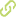# Optimality conditions for bilevel programming problems Chapter in Scopus•
• Overview
•
• Identity
•
•
• View All
•

### Abstract

• © 2006 Springer Science + Business Media, LLC.Focus in the paper is on optimality conditions for bilevel programming problems. We start with a general condition using tangent cones of the feasible set of the bilevel programming problem to derive such conditions for the optimistic bilevel problem. More precise conditions are obtained if the tangent cone possesses an explicit description as it is possible in the case of linear lower level problems. If the optimal solution of the lower level problem is a PC1-function, sufficient conditions for a global optimal solution of the optimistic bilevel problem can be formulated. In the second part of the paper relations of the bilevel programming problem to set-valued optimization problems and to mathematical programs with equilibrium constraints are given which can also be used to formulate optimality conditions for the original problem. Finally, a variational inequality approach is described which works well when the involved functions are monotone. It consists in a variational re-formulation of the optimality conditions and looking for a solution of the thus obtained variational inequality among the points satisfying the initial constraints. A penalty function technique is applied to get a sequence of approximate solutions converging to a solution of the original problem with monotone operators.

### Publication date

• January 1, 2006